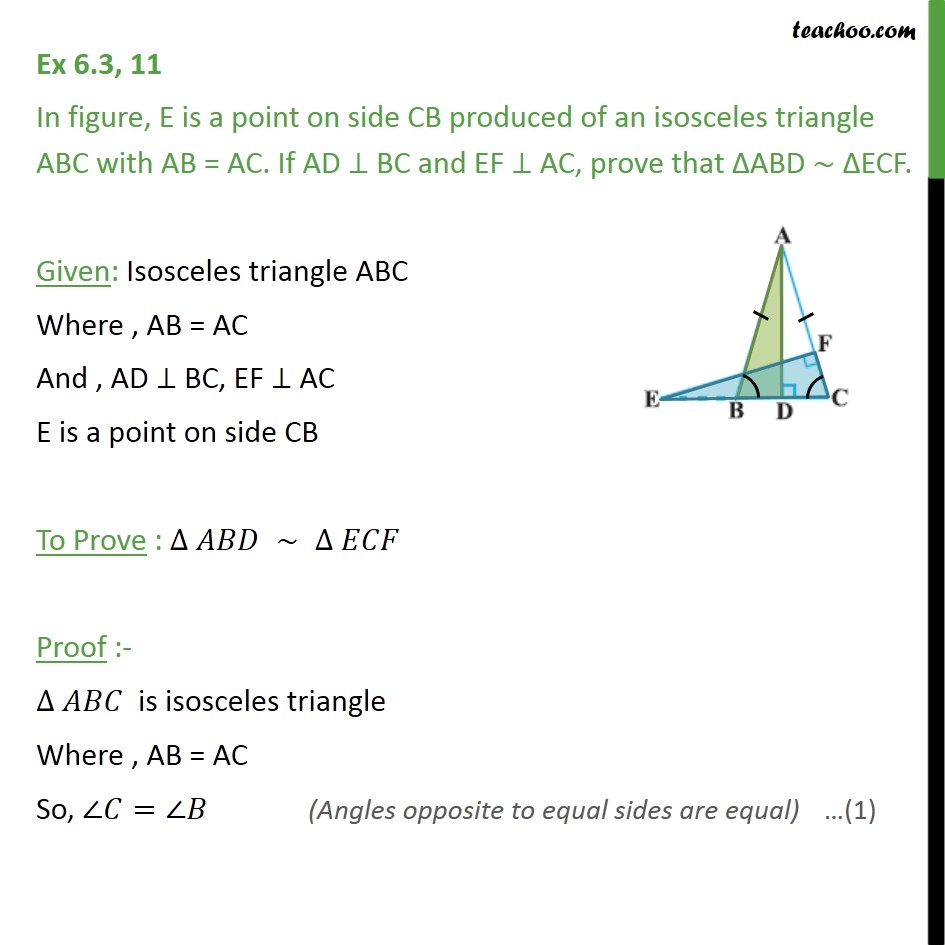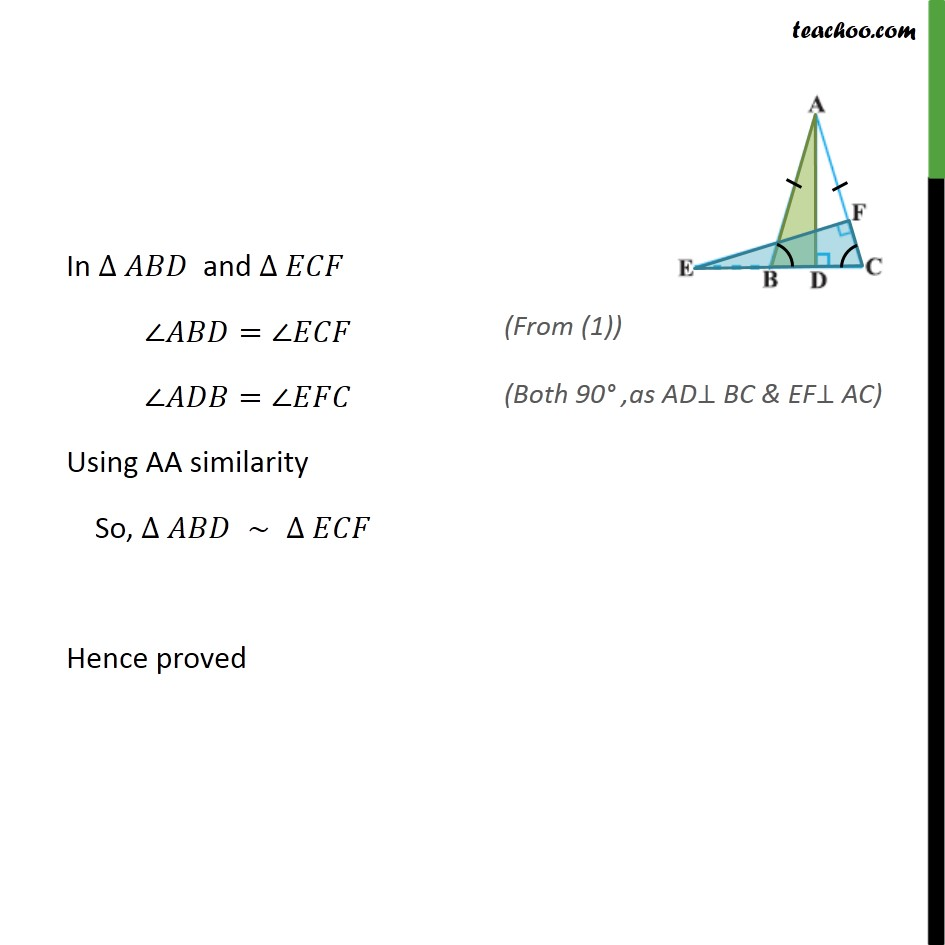Ex 6.3

Chapter 6 Class 10 Triangles
Serial order wiseLearn in your speed, with individual attention - Teachoo Maths 1-on-1 Class

### Transcript

Ex 6.3, 11 In figure, E is a point on side CB produced of an isosceles triangle ABC with AB = AC. If AD BC and EF AC, prove that ABD ECF. Given: Isosceles triangle ABC Where , AB = AC And , AD BC, EF AC E is a point on side CB To Prove : Proof :- is isosceles triangle Where , AB = AC So, = In and = = Using AA similarity So, Hence proved